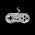Tuesday, January 6, 2015

Doppler Effect and Its Applications

The apparent change in the frequency due to the relative motion of source and observer is called Doppler Effect. We can experience the change in the frequency only when there is a relative motion. The original frequency of the source is not actually changing. Due to the relative motion it is appearing like changing and that’s why it is called as apparent change in frequency.

We can derive the equation for the apparent frequency in different possible cases. When the observer is in the motion he will receive more number of the waves than when he is in the state of rest. It is simply because waves are not only crossing him and he is also crossing the waves.

When the observer is crossing the stationary source there will be difference in the frequencies. If the observer is approaching the apparent frequency increases and when the observer is receding the apparent frequency decreases. The difference between these frequencies can be heard like beats to the observer. We can calculate the number of the beats as shown below.

There will be apparent change in the frequency even when the observer is the state of rest and the source is moving towards the Observer. Here is the source is approaching the observer, its wavelength towards the observer decreases and hence frequency increases. We can derive the equation for the apparent frequency in this case as shown below.

When the observer is in the state of the rest and source is approaching him, apparent frequency increases. When the source is moving away from the stationary observer, apparent frequency decreases. The difference between these two frequencies can be heard like the beats to the observer. We can derive the equation for the number of the beats as shown below.

When a source is revolving around the stationary observer, he is not going to have any Doppler effect experience. It is simply because there is no relative motion between the source and observer. No component of the velocity of the source is acting towards the observer and hence we cannot find any change in the frequency.

If the source is moving in the circular path and observer is far away from the Centre of the circular path, he can hear apparent frequency with different possible frequencies. When the source is moving away from the observer, apparent frequency decreases and vice versa. We can write the equation is as shown below.

When a source is moving by making an angle  to the direction of the observer, still there will be apparent change in the frequency due to the component of the velocity of the source towards Observer. We can write the equation for it as shown below.

Problem and solution

Problem and solution

Related Posts

Wave Motion an introduction

1 comment:

1.The frequency of a radar is 780Hz. The frequency of the reflected wave from an aeroplane is increased by 2.6Khz then what is the velocity of plane?????